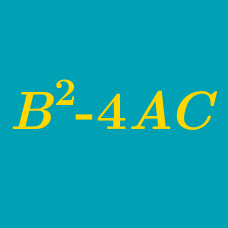Algebra

# Quadratic Discriminant - Range of Solutions

How many intersection points are there between the two graphs of the functions $y=2x^2-4x, y=3x+5?$

If real numbers $x$ and $y$ satisfy the equation $x^2+y^2-2x-14y+50=0,$ what is the value of $x+y$?

Consider two sets \begin{aligned} & A=\{(x,y) \mid y=x^2-2x+35\}, \\ & B=\{(x,y) \mid y=2kx-1\}. \end{aligned} How many integers $k$ satisfy $A \cap B=\emptyset$?

Find the values of $b$ such that $y=bx$ is a secant line to the curve $y=2x^2-b$.

Consider the graphs of $y=x^2+ax-5, y=2x-6.$ If they intersect at only one point, what is the value of the positive number $a$?

×